Next: Separation of the Nuclear Up: Molecular Quantum Mechanics Previous: The Molecular Hamiltonian

## The Born-Oppenheimer Approximation

We know that if a Hamiltonian is separable into two or more terms, then the total eigenfunctions are products of the individual eigenfunctions of the separated Hamiltonian terms, and the total eigenvalues are sums of individual eigenvalues of the separated Hamiltonian terms.

Consider, for example, a Hamiltonian which is separable into two terms, one involving coordinate q1 and the other involving coordinate q2.
(135)

with the overall Schrödinger equation being
(136)

If we assume that the total wavefunction can be written in the form
, where
and
are eigenfunctions of
and
with eigenvalues E1 and E2, then
=
(137) =
=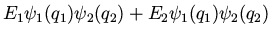=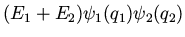=Thus the eigenfunctions of
are products of the eigenfunctions ofand, and the eigenvalues are the sums of eigenvalues ofand.

If we examine the nonrelativistic Hamiltonian (134), we see that the term
(138)

prevents us from cleanly separating the electronic and nuclear coordinates and writing the total wavefunction as, where
represents the set of all electronic coordinates, and
represents the set of all nuclear coordinates. The Born-Oppenheimer approximation is to assume that this separation is nevertheless approximately correct.

Qualitatively, the Born-Oppenheimer approximation rests on the fact that the nuclei are much more massive than the electrons. This allows us to say that the nuclei are nearly fixed with respect to electron motion. We can fix, the nuclear configuration, at some value
, and solve for
; the electronic wavefunction depends only parametrically on. If we do this for a range of, we obtain the potential energy curve along which the nuclei move.

We now show the mathematical details. Let us abbreviate the molecular Hamiltonian as
(139)

where the meaning of the individual terms should be obvious. Initially,
can be neglected since
is smaller than
by a factor of MA / me, where me is the mass of an electron. Thus for a fixed nuclear configuration, we have
(140)

such that
(141)

This is the clamped-nuclei'' Schrödinger equation. Quite frequently
is neglected in the above equation, which is justified since in this caseis just a parameter so thatis just a constant and shifts the eigenvalues only by some constant amount. Leavingout of the electronic Schrödinger equation leads to a similar equation,
(142)
(143)

where we have used a new subscript e'' on the electronic Hamiltonian and energy to distinguish from the case whereis included.

We now consider again the original Hamiltonian (139). If we insert a wavefunction of the form
, we obtain
(144)(145)

Sincecontains nodependence,
(146)

However, we may not immediately assume
(147)

(this point is tacitly assumed by most introductory textbooks). By the chain rule,(148)

Using these facts, along with the electronic Schrödinger equation,
(149)

we simplify (145) to
(150)
=
We must now estimate the magnitude of the last term in brackets. Following Steinfeld , a typical contribution has the form
, but
is of the same order as
since the derivatives operate over approximately the same dimensions. The latter is
, with pe the momentum of an electron. Therefore. Since
, the term in brackets can be dropped, giving(151)
(152)

This is the nuclear Shrodinger equation we anticipated--the nuclei move in a potential set up by the electrons.

To summarize, the large difference in the relative masses of the electrons and nuclei allows us to approximately separate the wavefunction as a product of nuclear and electronic terms. The electronic wavefucntion
is solved for a given set of nuclear coordinates,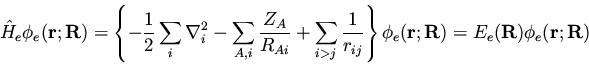(153)

and the electronic energy obtained contributes a potential term to the motion of the nuclei described by the nuclear wavefunction
.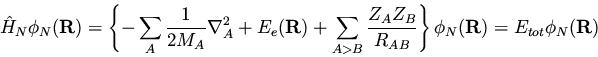(154)

As a final note, many textbooks, including Szabo and Ostlund , mean total energy at fixed geometry when they use the term total energy'' (i.e., they neglect the nuclear kinetic energy). This is just Eel of equation (141), which is also Ee plus the nuclear-nuclear repulsion. A somewhat more detailed treatment of the Born-Oppenheimer approximation is given elsewhere .Next: Separation of the Nuclear Up: Molecular Quantum Mechanics Previous: The Molecular Hamiltonian